Warning: this document is still work in progress. Feel free to send comments but do not consider it final material.

## 2. Halftoning

### 2.1. Halftoning patterns

Observe the following patterns. From a certain distance or assuming small enough pixels, they look like shades of grey despite being made of only black and white pixels: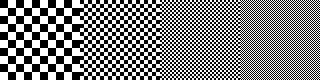We can do even better using additional patterns such as these 25% and 75% halftone patterns: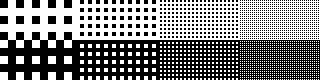This looks promising. Let’s try immediately on Lena: we will use the 5-colour thresholding picture and replace the 0.25, 0.5 and 0.75 grey values with the above patterns: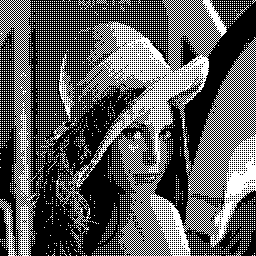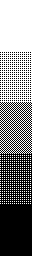Not bad for a start. But there is a lot to improve. By the way, this technique is covered by Apple’s U.S. patent 5761347 .

### 2.2. Screen artifacts

If your screen’s quality is not very good, you might experience slightly different shades of grey for the following patterns, despite being made of 50% black and 50% white pixels: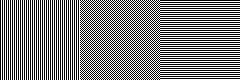Obviously the middle pattern looks far better to the human eye on a computer screen. Optimising patterns so that they look good to the human eye and don't create artifacts is a crucial element of a dithering algorithm. Here is another example of two patterns that approximate to the same shade of grey but may look slightly different from a distance: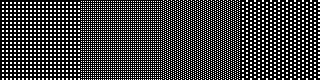### 2.3. Ordered dithering

A generalisation of the dithering technique we just saw that uses a certain family of patterns is called ordered dithering. It is based on a dither matrix such as the following one: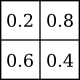Using the matrix coefficients as threshold values yield the following results for black, white and three shades of grey (0.25, 0.5 and 0.75):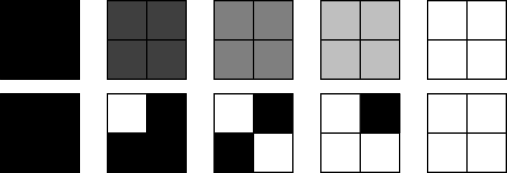The dither matrix is therefore repeated all over the image. The first pixel will be thresholded with a value of 0.2, the second pixel with a value of 0.8, then the third pixel with a value of 0.2 again, and so on, resulting in an image very similar to the one previously seen in 2.1: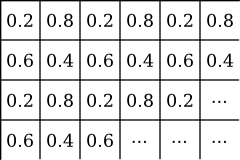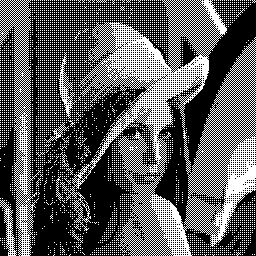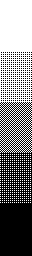For better readability, the matrix is rewritten as following. The dither coefficients are trivially computed from the matrix cells and the matrix size: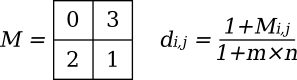Different matrices can give very different results. This is a 4×4 Bayer ordered dither matrix , recursively created from the previous 2×2 dither matrix: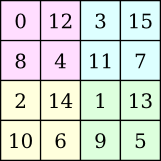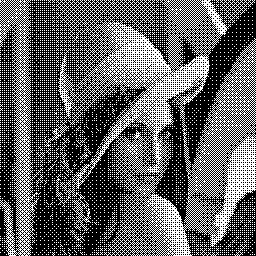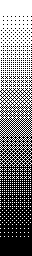This is an 8×8 Bayer matrix, recursively created from the 4×4 version: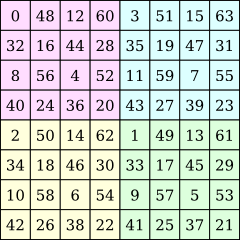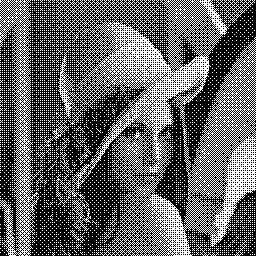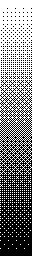This 4×4 cluster dot matrix creates dot patterns: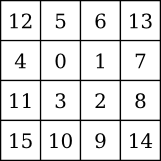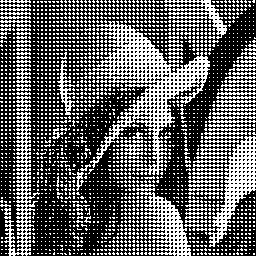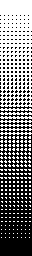This 8×8 cluster dot matrix mimics the halftoning techniques used by newspapers: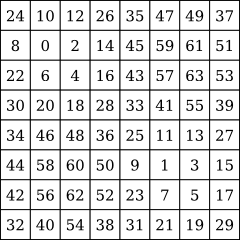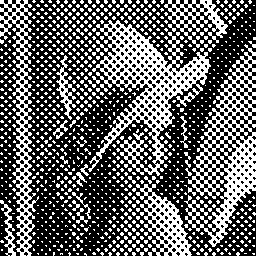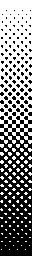This unusual 5×3 matrix creates artistic vertical line artifacts: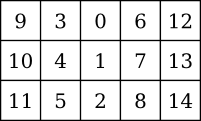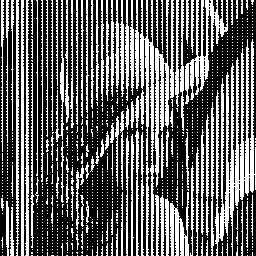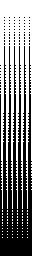There are two major issues with ordered dithering. First, important visual artifacts may appear. Even Bayer ordered dithering causes weird cross-hatch pattern artifacts on some images. Second, dithering matrices do not depend on the original image and thus do not take input data into account: high frequency features in the image are often missed and, in some cases, cause even worse artifacts.

### 2.4. Random ordered dithering

Random dithering can help reduce the major problem caused by halftoning, which is the apparition of pattern artifacts. The method is as simple as slightly perturbating dither matrix coefficients (or pixel values) during the halftoning step. The difficult part is picking up an adequate perturbation function: too much perturbation and the result is unrecognisable, too little and the artifacts stay.

For instance, this is the result of 8×8 Bayer dithering perturbated by a gaussian distribution (mean 0.0, standard deviation 0.08):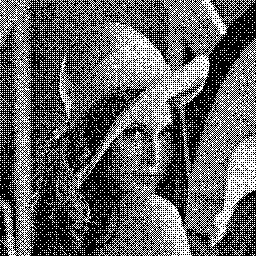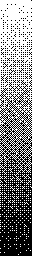Another way to use random number generators to avoid pattern artifacts is random dither matrix selection . The image space is no longer tiled with the same matrix over and over again, but with a random selection from a list of similar dither matrices.

This example shows random matrix selection from a list of six 3×3 dither matrices: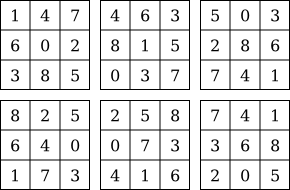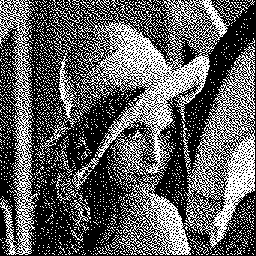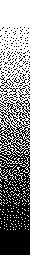### 2.5. Non-rectangular dither tiles

Another way to avoid disturbing pattern artifacts is to use non-rectangular dither tiles. Here are several examples, the first one generating slanted square patterns, the second one hexagonal patterns, then slanted square patterns again with a slightly different angle, and hexagonal patterns again. The artifacts usually seen in Bayer dithering do not appear here: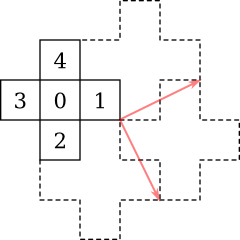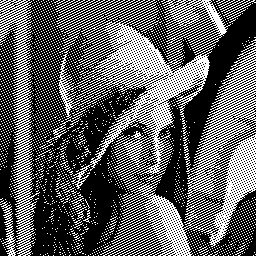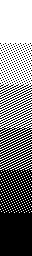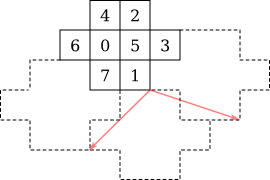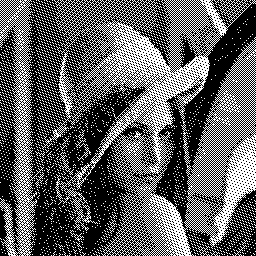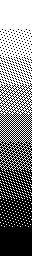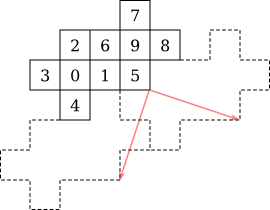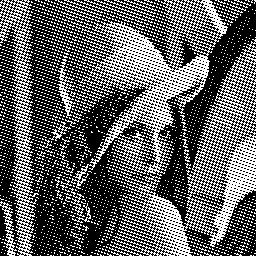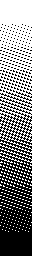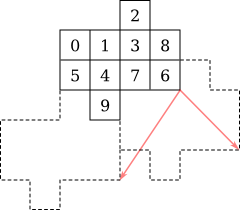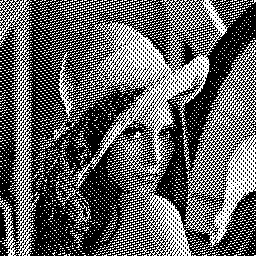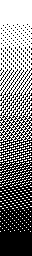### 2.6. Supercell dithering

Supercell dithering consists in creating bigger dithering tiles (supercells) from base tiles. One example is Victor Ostromoukhov’s CombiScreen method .

Just like Bayer matrices, non-rectangular tiles can be used to recursively create bigger patterns, giving finer results. The amount of shades of grey that can be rendered using a given tile is the number of cells in the tile plus one. Here are a few examples using tiles seen previously: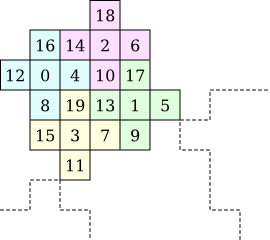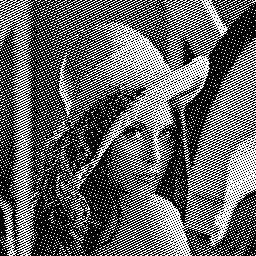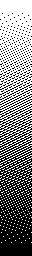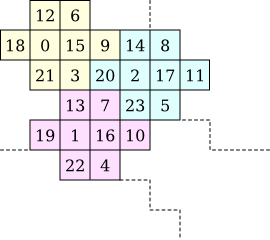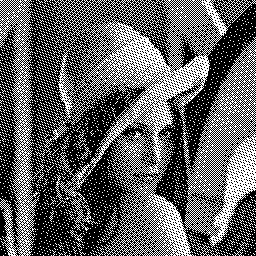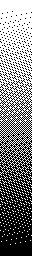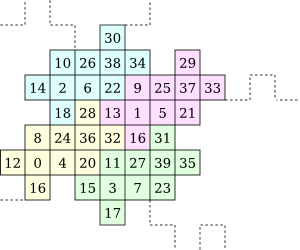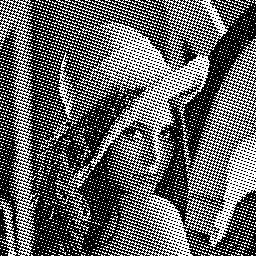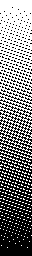This example shows a tile resembling a Davis-Knuth dragon curve. Though the tile itself is beautiful, it is in reality only a reorganisation of an 8×8 Bayer dither matrix. Therefore the resulting image is exactly the same as for classical Bayer dithering: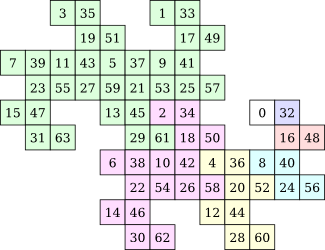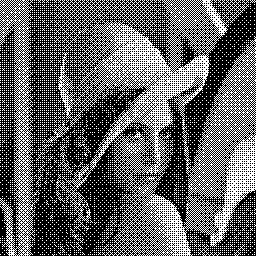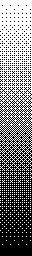Here are two consecutive iterations of the hexagonal tiling shown above. Since the area of the original tile is 10 cells, the first iteration could display 11 different shades of grey. These iterations can display respectively 31 and 91 shades: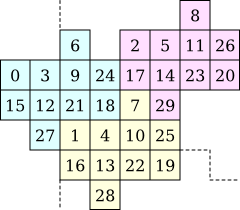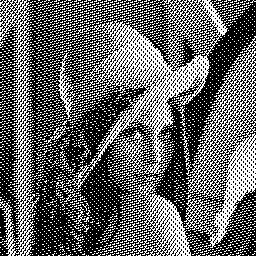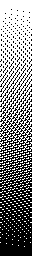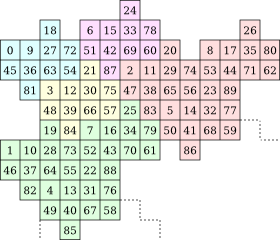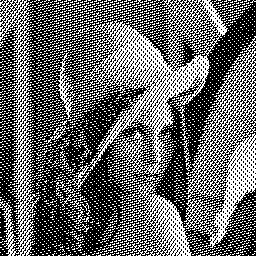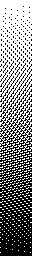### 2.7. Void and cluster method

Robert A. Ulichney’s void and cluster method  is a very generic method for dither array generation. It mainly targets huge matrices in order to reduce artifacts caused by tiling.

The process goes through many steps. First, a working pattern matrix needs to be created:

• Generate an empty w×h matrix full of 0s
• Set n cells to 1
• The working matrix is uniformly distributed:
• Find the 1 with the most neighbours set to 1 and set that cell to 0
• Find the 0 with the fewest neighbours set to 1 and set that cell to 1
• Repeat until the 1 was just put back where it originally was

Usually n is about 10% of w×h. It can be generated randomly, loaded from a known pattern, or created using a more powerful algorithm (chapter 3 will introduce error diffusion algorithms that may be used to generate the working pattern matrix).

The dither matrix is then generated from the working pattern in three steps:

• Dither indices 0 to n - 1 are set using a first copy of the working matrix
• n = n - 1
• Find the 1 with the most neighbours set to 1 and set that cell to 0
• In the dither matrix, set the corresponding cell to n
• Repeat until n = 0
• Dither indices n to w×h/2 are set using a second copy of the working matrix
• Find the 0 with the fewest neighbours set to 1 and set that cell to 1
• In the dither matrix, set the corresponding cell to n
• n = n + 1
• Repeat until n ≥ w×h/2
• Dither indices w×h/2 to w×h are set using the same copy of the working matrix
• Find the 0 with the fewest neighbours set to 1 and set that cell to 1
• In the dither matrix, set the corresponding cell to n
• n = n + 1
• Repeat until n = w×h

The key part of the algorithm is the choice of the void finder and the cluster finder for each step. Best results are achieved using Voronoï tesselation  , but simpler methods such as gaussian convolution  give decent results, too.

The following two matrices show the results of the algorithm using randomly generated initial matrices of size respectively 14×14 and 25×25. The void and cluster finder uses a simple 7×7 gaussian convolution filter. Gray cells show the initial uniformly distributed matrix: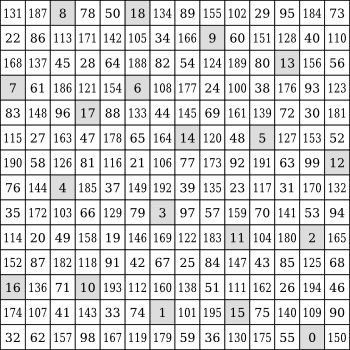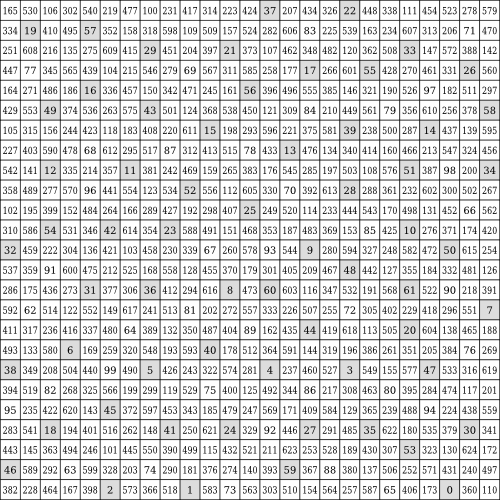Dither matrices generated with the void and cluster method give impressive results. They are pretty close to the best quality that can be achieved using standard ordered dithering: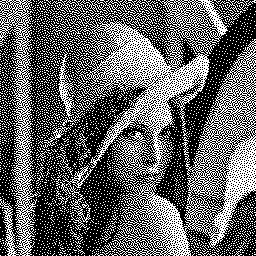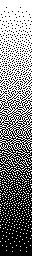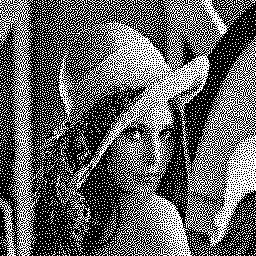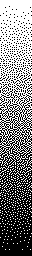This technique is covered by Ulichney’s U.S. patent 5535020 and the specific implementation we showed is partly covered by Epson’s U.S. patent 6088512.